### Conditions of Optimality

#### Linear Programming

A standard linear program has the following formulation: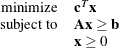where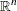is the vector of decision variables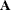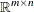is the matrix of constraintsis the vector of objective function coefficients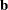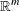is the vector of constraints right-hand sides (RHS)

This formulation is called the primal problem. The corresponding dual problem (see the section Dual Values) is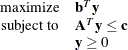where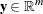is the vector of dual variables.

The vectorsandare optimal to the primal and dual problems, respectively, only if there exist primal slack variables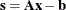and dual slack variables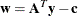such that the following Karush-Kuhn-Tucker (KKT) conditions are satisfied: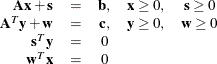The first line of equations defines primal feasibility, the second line of equations defines dual feasibility, and the last two equations are called the complementary slackness conditions.

#### Nonlinear Programming

To facilitate discussion of optimality conditions in nonlinear programming, you write the general form of nonlinear optimization problems by grouping the equality constraints and inequality constraints. You also write all the general nonlinear inequality constraints and bound constraints in one form as
inequality constraints. Thus, you have the following formulation: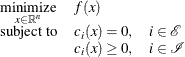where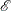is the set of indices of the equality constraints,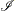is the set of indices of the inequality constraints, and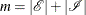.

A pointis feasible if it satisfies all the constraints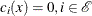and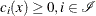. The feasible region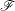consists of all the feasible points. In unconstrained cases, the feasible regionis the entirespace.

A feasible point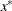is a local solution of the problem if there exists a neighborhood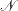ofsuch that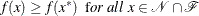Further, a feasible pointis a strict local solution if strict inequality holds in the preceding case; that is,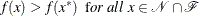A feasible pointis a global solution of the problem if no point inhas a smaller function value than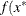); that is,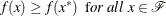##### Unconstrained Optimization

The following conditions hold true for unconstrained optimization problems:

• First-order necessary conditions:  Ifis a local solution and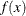is continuously differentiable in some neighborhood of, then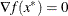• Second-order necessary conditions:  Ifis a local solution andis twice continuously differentiable in some neighborhood of, then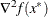is positive semidefinite.

• Second-order sufficient conditions:  Ifis twice continuously differentiable in some neighborhood of,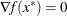, andis positive definite, thenis a strict local solution.

##### Constrained Optimization

For constrained optimization problems, the Lagrangian function is defined as follows: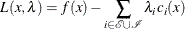where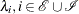, are called Lagrange multipliers.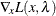is used to denote the gradient of the Lagrangian function with respect to, and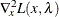is used to denote the Hessian of the Lagrangian function with respect to. The active set at a feasible pointis defined as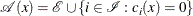You also need the following definition before you can state the first-order and second-order necessary conditions:

• Linear independence constraint qualification and regular point:  A pointis said to satisfy the linear independence constraint qualification if the gradients of active constraints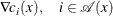are linearly independent. Such a pointis called a regular point.

You now state the theorems that are essential in the analysis and design of algorithms for constrained optimization:

• First-order necessary conditions:  Suppose thatis a local minimum and also a regular point. Ifand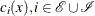, are continuously differentiable, there exist Lagrange multipliers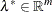such that the following conditions hold: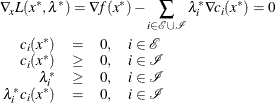The preceding conditions are often known as the Karush-Kuhn-Tucker conditions, or KKT conditions for short.

• Second-order necessary conditions:  Suppose thatis a local minimum and also a regular point. Let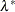be the Lagrange multipliers that satisfy the KKT conditions. Ifand, are twice continuously differentiable, the following conditions hold: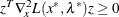for all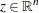that satisfy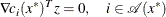• Second-order sufficient conditions:  Suppose there exist a pointand some Lagrange multiplierssuch that the KKT conditions are satisfied. If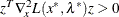for allthat satisfythenis a strict local solution.

Note that the set of all such’s forms the null space of the matrix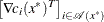. Thus, you can search for strict local solutions by numerically checking the Hessian of the Lagrangian function projected onto the null space. For a rigorous treatment of the optimality conditions, see Fletcher (1987) and Nocedal and Wright (1999).